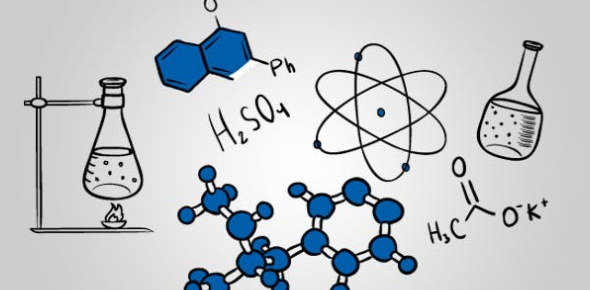# Chemistry Unit Test For Grade 9

19 Questions | Total Attempts: 10809SettingsChemistry is the branch of science which deals specifically with the elements on the periodic table, as well as compounds, all of which are composed of atoms, molecules, and ions. In this quiz, we aim to boost your knowledge on a chemistry-oriented level on some everyday items such as salt and aluminum foil, as well as your expertise on individual elements and their chemical symbol.

Related Topics
• 1.
Which of the following are four primary indicators of a physical change?
• A.

Color change

• B.

Luster

• C.

Malleability

• D.

Energy change

• E.

Optical clarity

• F.

Electrical conductivity

• 2.
Which of the following are four primary indicators of a chemical change?
• A.

Color change

• B.

Odor

• C.

Ductility

• D.

Gas production

• E.

Viscosity

• F.

Energy change

• 3.
Name the element- Na
• A.

Sodium

• B.

Nitrogen

• C.

Sulfur

• 4.
Name the element- K
• A.

Hydrogen

• B.

Sulphur

• C.

Potassium

• 5.
Name the element- S
• A.

Silver

• B.

Sulphur

• C.

Sodium

• 6.
Name the element- Li
• A.

Beryllium

• B.

Lithium

• C.

Calcium

• 7.
Protium, Deuterium, and Tritium are all isotopes of:
• 8.
The formulas for density are:
• A.

D= m/v

• B.

D= m + v

• C.

M= D - v

• D.

M= Dv

• E.

V= Dm

• F.

V= m/D

• 9.
P+ means the number of protons.
• A.

True

• B.

False

• 10.
"N" means the number of neutrons.
• A.

True

• B.

False

• 11.
E- means the number of electrons.
• A.

True

• B.

False

• 12.
How many electrons can there be in ring #1 in a Bohr- rutherford diagram?
• A.

2

• B.

4

• C.

6

• D.

1

• E.

3

• 13.
What is an ionic compound?
• A.

An element that is a metal and a non-metal.

• B.

Forms between a metal and a non-metal. The metal gives its electrons to the non-metal.

• C.

An electron (e-)

• 14.
Magnesium has an atomic weight of 24. What is it's isotope name?
• A.

Magnesium- 24.3

• B.

Magnesium- 23

• C.

Magnesium- 24

• D.

Magnesium- 24.3

• 15.
Nitrogen has a rounded atomic weight of 15. What is it's isotope name?
• A.

Nitrogen-14.59

• B.

Nitrogen- 15

• C.

Nitrogen- 150

• 16.
What is Mg3P2 called?
• A.

Magnesium Phosphorus

• B.

Magnesium Phosphite

• C.

Magnesium phosphide

• 17.
What is Sodium Chloride called?
• A.

Sodium Chlorate

• B.

Sodium Chloride

• C.

Sodiumide Chloride

• 18.
How do you present Calcium iodide?
• A.

CaI

• B.

CaI2

• C.

CaII

• 19.
How do you represent Lithium Chloride?
• A.

LiCl

• B.

LICL

• C.

ClLi